## RD Sharma Class 9 Solutions Chapter 5 Factorisation of Algebraic Expressions Ex 5.1

These Solutions are part of RD Sharma Class 9 Solutions. Here we have given RD Sharma Class 9 Solutions Chapter 5 Factorisation of Algebraic Expressions Ex 5.1

Other Exercises

Factorize
Question 1.
x3 + x – 3x2 – 3
Solution:
x3 + x – 3x2 – 3
x3 – 3a2 + x – 3
⇒  x2(x – 3) + 1(x – 3)
= (x – 3) (x2 + 1)

Question 2.
a(a + b)3 – 3a2b(a + b)
Solution:
a(a + b)3 – 3a2b(a + b)
= a(a + b) {(a + b)2 3ab}
= a(a + b) {a2 + b2 + 2ab – 3ab}
= a{a + b) {a2 – ab + b2)

Question 3.
x(x3 – y3) + 3xy(x – y)
Solution:
x(x3 – y3) + 3xy(x – y)
= x(x – y) (x2 + xy + y2) + 3xy(x – y)
= x(x – y) (x2 + xy + y2 + 3y)
= x(x – y) (x2 + xy + y2 + 3y)

Question 4.
a2x2 + (ax2 +1)x + a
Solution:
a2x2 + (ax2 + 1)x + a
= a2x2 + a + (ax2 + 1)x
= a(ax2 + 1) + x(ax2 + 1)
= (ax2 + 1) (a + x)
= (x + a) (ax2 + 1)

Question 5.
x2 + y – xy – x
Solution:
x2 + y – xy – x
= x2-x-xy + y = x(x- l)-y(*- 1)
= (x – 1) (x – y)

Question 6.
X3 – 2x2y + 3xy2 – 6y3
Solution:
x3 – 2x2y + 3xy26y3
= x2(x – 2y)
+ 3y2(x – 2y)
= (x – 2y) (x2 + 3y2)

Question 7.
6ab – b2 + 12ac – 2bc
Solution:
6ab – b2 + 12ac – 2bc
= 6ab + 12ac – b2 – 2bc
= 6a(b + 2c) – b(b + 2c)
= (b + 2c) (6a – b)

Question 8.
x(x – 2) (x – 4) + 4x – 8
Solution:
x(x – 2) (x – 4) + 4x – 8
= x(x – 2) (x – 4) + 4(x – 2)
= (x – 2) [x(x – 4) + 4]
= (x – 2) (x2 – 4x + 4)
= (x – 2) [(x)2 – 2 x x x 2 + (2)2]
= (x – 2) (x – 2)2 = (x – 2)3

Question 9.
(a – b + c)2 + (b – c + a)2 + 2(a – b + c) (b – c + a)
Solution:
(a – b + c)2 + ( b- c+a)2 + 2(a – b + c) (b – c + a)      {∵ a2 + b2 + 2ab = (a + b)2}
= [a – b + c + b- c + a]2
= (2a)2 = 4a2

Question 10.
a2 + 2ab + b2 – c2
Solution:
a2 + 2ab + b2 – c2
= (a2 + 2ab + b2) – c2
= (a + b)2 – (c)2         {∵  a2 – b2 = (a + b) (a – b)}
= (a + b + c) (a + b – c)

Question 11.
a2 + 4b2 – 4ab – 4c2
Solution: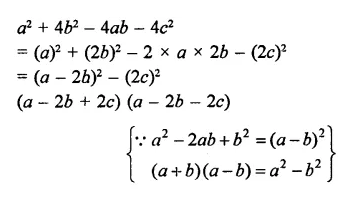Question 12.
x2 – y2 – 4xz + 4z2
Solution:
x2 – y2 – 4xz + 4z2
= x2 – 4xz + 4z2 – y2
= (x)2 – 2 x x x 2z + (2z)2 – (y)2
= (x – 2z)2 – (y)2
= (x – 2z + y) (x – 2z – y)
= (x +y – 2z) (x – y – 2z)

Question 13.Solution: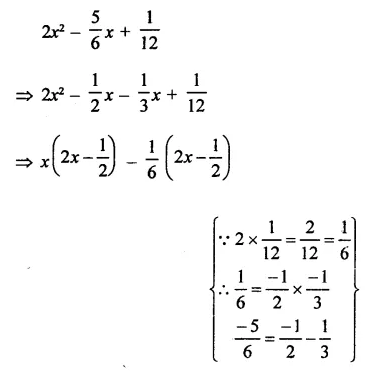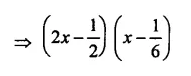Question 14.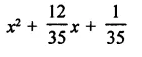Solution: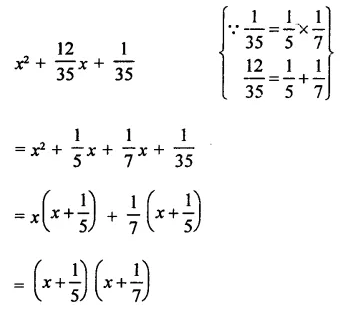Question 15.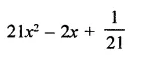Solution:Question 16.
Give possible expression for the length and breadth of the rectangle having 35y2 + 13y – 12 as its area.
Solution:
Area of a rectangle = 35y2 + 13y – 12
= 35y2 + 28y- 15y- 12(i) If length = 5y + 4, then breadth = 7y – 3
(ii) and if length = 7y-3, then length = 5y+ 4

Question 17.
What are the possible expressions for the dimensions of the cuboid whose volume is 3x2 – 12x.
Solution:
Volume 3x2 – 12x
= 3x(x – 4)
∴ Factors are 3, x, and x – 4
Now, if length = 3, breadth = x and height = x – 4
if length =3, breadth = x – 4, height = x
if length = x, breadth = 3, height = x – 4
if length = x, breadth = x – 4, height = 3
if length = x – 4, breadth = 3, height = x
if length – x – 4, breadth = x, height = 3

Question 18.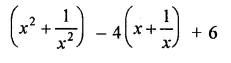Solution: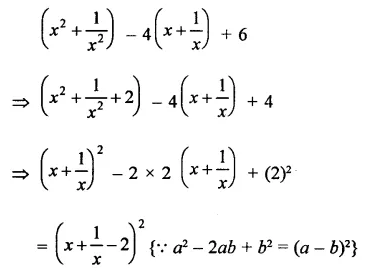Question 19.
(x + 2) (x2 + 25) – 10x2 – 20x
Solution:
(x + 2) (x2 + 25) – 10x2 – 20x
= (x + 2) (x2 + 25) – 10x(x + 2)
= (x + 2) [x2 + 25 – 10x]
= (x + 2) [(x)2 – 2 x x x 5 + (5)2]
= (x + 2) (x – 5)2

Question 20.
2a2 + 2$$\sqrt { 6 }$$ ab +3b2
Solution:
2a2 + 2$$\sqrt { 6 }$$  ab +3 b2
= ($$\sqrt { 2 }$$ a)2+ $$\sqrt { 2 }$$ a x $$\sqrt { 3 }$$ b+ ($$\sqrt { 3 }$$ b)2
= ($$\sqrt { 2 }$$a + $$\sqrt { 3 }$$ b)2

Question 21.
a2 + b2 + 2(ab + bc + ca)
Solution:
a2 + b2 + 2(ab + bc + ca)
= a2 + b2 + 2 ab + 2 bc + 2 ca
= (a + b)2 + 2c(b + a)
= (a + b)2 + 2c(a + b)
= (a + b) (a + b + 2c)

Question 22.
4(x – y)2 – 12(x -y) (x + y) + 9(x + y)2
Solution:
4(x – y)2 – 12(x – y) (x + y) + 9(x + y)2
= [2(x – y)2 + 2 x 2(x – y) x 3(x + y) + [3 (x+y]2        { a2 + b2 + 2 abc = (a + b)2}
= [2(x – y) + 3(x + y)]2
= (2x-2y + 3x + 3y)2
= (5x + y)2

Question 23.
a2 – b2 + 2bc – c2
Solution:
a2 – b2 + 2bc – c2
= a2 – (b2 – 2bc + c2)                                           { a2 + b2 – 2abc = (a – b)2}
= a2 – (b – c)2
= (a)2 – (b – c)2          { a2 – b2 = (a + b) (a – b)}
= (a + b – c) (a – b + c)

Question 24.
xy9 – yx9
Solution:
xy9 – yx9 = xy(y8 – x8)
= -xy(x8 – y8)
= -xy[(x4)2 – (y4)2]
= -xy (x4 + y4) (x4 – y4)                                         {∵ a2-b2 = (a + b) (a – b)}
= -xy (x4 + y4) {(x2)2 – (y2)2}
= -xy(x+ y4) (x2 + y2) (x2 – y2)
= -xy (x4 +y4) (x2 + y2) (x + y) (x -y)
= -xy(x – y) (x + y) (x2 + y2) (x4 + y4)

Question 25.
x4 + x2y2 + y4
Solution:
x4 + x2y2 + y4  = (x2)2 + 2x2y2 + y4 – x2y2           (Adding and subtracting x2y2)
= (x2 + y2)2 – (xy)2                                                        { a2 – b2 = (a + b) (a – b)}
= (x2 + y2 + xy) (x2 + y2 – xy)
= (x2 + xy + y2) (x2 – xy + y2)

Question 26.
x2 + 6$$\sqrt { 2 }$$x + 10
Solution: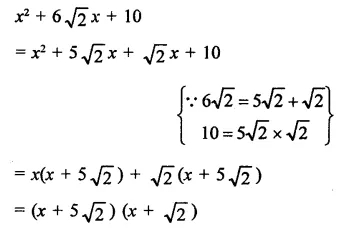Question 27.
x2 + 2$$\sqrt { 2 }$$x- 30
Solution: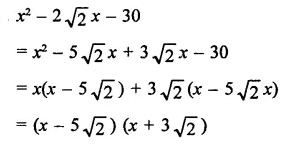Question 28.
x2 – $$\sqrt { 3 }$$x – 6
Solution:Question 29.
x2 + 5 $$\sqrt { 5 }$$x + 30
Solution:Question 30.
x2 + 2 $$\sqrt { 3 }$$x – 24
Solution:Question 31.
5 $$\sqrt { 5 }$$x2 + 20x + 3$$\sqrt { 5 }$$
Solution:Question 32.
2x2 + 3$$\sqrt { 5 }$$ x + 5
Solution: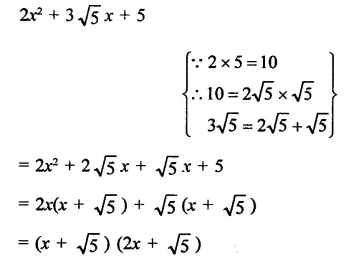Question 33.
9(2a – b)2 – 4(2a – b) – 13
Solution: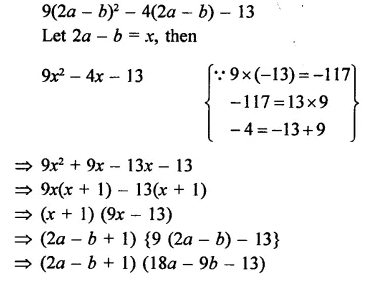Question 34.
7(x-2y) – 25(x-2y) +12
Solution: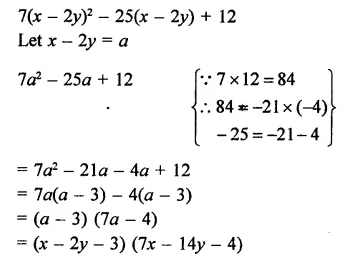Question 35.
2(x+y) – 9(x+y) -5
Solution:
2(x+y) – 9(x+y) -5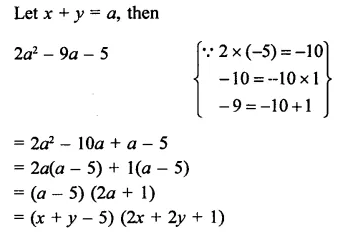Hope given RD Sharma Class 9 Solutions Chapter 5 Factorisation of Algebraic Expressions Ex 5.1 are helpful to complete your math homework.

If you have any doubts, please comment below. Learn Insta try to provide online math tutoring for you.